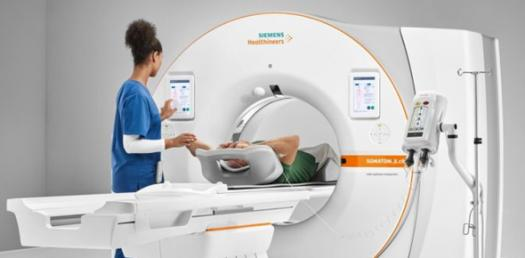# Final Test CT Scan/ Anatomy

50 Questions | Total Attempts: 678Settings.

• 1.
The main purpose of the slip ring technology is to:
• A.

Increase the number of dectors that can be housed in the gantry

• B.

Make the CT scanning operation quieter

• C.

Allow for continous rotation of the dectors and X-ray tube around the patient

• D.

Reduce the potential for motion artifacts associated with spiral/ helical scanning

• 2.
The CT number for water is:
• A.

Varies depending on the type of tissue being scanned

• B.

Should always be near 0

• C.

Is irrelevent to CT scanning

• D.

Needs to be factored into tissues containing alot of water

• 3.
____________, pick up and measure the radiation passing through a cross-section of the patiant, convert the transmitted photons into an electrical signal, and then send the signal to the computer system for processing.
• A.

Collimators

• B.

Detectors

• C.

Filters

• D.

Slip Rings

• 4.
• A.

• B.

• C.

• D.

• 5.
There are ____ cervical vertebrae?
• A.

5

• B.

6

• C.

7

• D.

8

• 6.
The __________ axix is referred to as the sagittal plane.
• A.

X axis

• B.

Y axis

• C.

Z axis

• D.

None of the above

• 7.
CT scanners contain what type of collimators:
• A.

Pre patient collimators

• B.

Post patient collimators

• C.

Both pre and post patient collimators

• D.

Automatic collimators

• 8.
Thin collimation results in ________ resolution:
• A.

Less

• B.

Better

• C.

Both

• 9.
The scan field of view (SFOV) is selected _______ the scan begains
• A.

After

• B.

Before

• C.

During

• 10.
The display field of view (DFOV) can be equal to or ________ than the scan field of view?
• A.

Less than

• B.

Greater than

• C.

Same size as the SFOV

• 11.
The ________ axis, or the coronal plane divides the patiants body into anterior and posterior sections.
• A.

X axis

• B.

Y axis

• C.

Z axis

• D.

A axis

• 12.
Spatial resolution describes the degree of blurring in an image and is a measure of how small an object can be imaged.
• A.

True

• B.

False

• 13.
Preprocessed CT data is referrred to as_____
• A.

Raw data

• B.

Image Data

• C.

Display data

• D.

Scan data

• 14.
Reconstruction that is performed at a much later time is known as________;
• A.

Multiplaner reconstruction

• B.

Curved reconstruction

• C.

Minimum intensity projection

• D.

Retrospective reconstruction

• 15.
The Z axis is called the _______ because it divides the body into superior and inferior parts.
• A.

Coronal Plane

• B.

Z gap

• C.

Axial Plane

• D.

Image Plane

• 16.
Acute renal faliure occurs after all IV contrast injections
• A.

True

• B.

False

• 17.
CT contrast can be administered via:
• A.

PO, Oral, and Rectal

• B.

Oral, Po, and IV

• C.

Iv, Oral, and Rectal

• D.

Rectal, PO, and oral

• 18.
A patient with an allergy to Iodine can still be injected after premedication has been adminstered.
• A.

True

• B.

False

• 19.
The ______houses most of the functional equipment parts needed to acquire image data.
• A.

Generator

• B.

Computer

• C.

Gantry

• D.

Table

• 20.
Cardiac arrest is classified as a _______reaction:
• A.

Severe

• B.

Minor

• C.

Intermediate

• D.

Death

• 21.
The detectors which measure the patiants attenuation data are located _______ the XRay tube.
• A.

Inside

• B.

Under

• C.

Next to

• D.

Opposite

• 22.
As the pitch increases the patient moves through the gantry at a _____ rate.
• A.

Faster

• B.

Slower

• C.

Table speed does not change

• 23.
As the pitch increases the patiant dose ______.
• A.

Doubles

• B.

Increases

• C.

Stays the same

• D.

Decreases

• 24.
Window width (WW) determines the maximun ________ that can be displayed on the CT monitor.
• A.

Pixels

• B.

Number of Images

• C.

• D.

Size of matrix

• 25.
A pixel is a ________ element that is assigned a gray value in the form of a ct number.
• A.

Box

• B.

Two- dimensional

• C.

3D

• D.

Small

Related TopicsBack to top